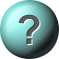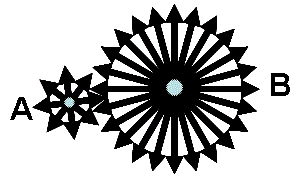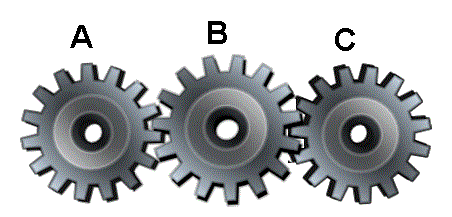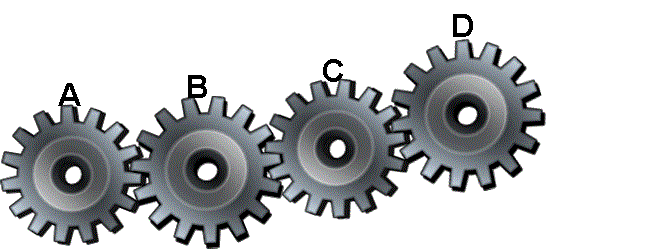### Mechanical Aptitude 3 - Gears1.    If the driven gear has 24 teeth and the driver gear has 8, then the driven gear?Turns at same speed as of driver gearTurns three times slower than the driverTurns three times faster than the driverActually driver gear moves slower2.    For gear ratio of 3 - to - 1, if input torque is 100 Newton Meters ( unit of torque measurement in the SI system) then output torque is?1/3 times i.e. 33.33 Newton Meters.3 times i.e. 300 Newton Meters.It has no effect on output torque.Two answers are correct3.    When three gears are in mesh, the central gear (B) is called?Connecting gearIntermediate gearIdler gearTwo answers are correct4.    The idler gear transfers’ movement between the input and output gears, and?Has no effect on the ratioHas no effect on the torque multiplicationTorque and ratio remain unchangedAll answers are correct5.    Adjacent Gears always move in__________?ClockwiseOppositeSameAnti clockwise

This is more feedback!
This is the feedback!